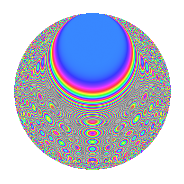# Properties

 Label 630.2.btLevel 630 Weight 2 Character orbit bt Rep. character $$\chi_{630}(317,\cdot)$$ Character field $$\Q(\zeta_{12})$$ Dimension 192 Newforms 1 Sturm bound 288 Trace bound 0

# Related objects

## Defining parameters

 Level: $$N$$ = $$630 = 2 \cdot 3^{2} \cdot 5 \cdot 7$$ Weight: $$k$$ = $$2$$ Character orbit: $$[\chi]$$ = 630.bt (of order $$12$$ and degree $$4$$) Character conductor: $$\operatorname{cond}(\chi)$$ = $$315$$ Character field: $$\Q(\zeta_{12})$$ Newforms: $$1$$ Sturm bound: $$288$$ Trace bound: $$0$$

## Dimensions

The following table gives the dimensions of various subspaces of $$M_{2}(630, [\chi])$$.

Total New Old
Modular forms 608 192 416
Cusp forms 544 192 352
Eisenstein series 64 0 64

## Trace form

 $$192q$$ $$\mathstrut +\mathstrut 8q^{6}$$ $$\mathstrut +\mathstrut O(q^{10})$$ $$192q$$ $$\mathstrut +\mathstrut 8q^{6}$$ $$\mathstrut +\mathstrut 12q^{15}$$ $$\mathstrut +\mathstrut 96q^{16}$$ $$\mathstrut +\mathstrut 36q^{17}$$ $$\mathstrut +\mathstrut 16q^{18}$$ $$\mathstrut -\mathstrut 12q^{21}$$ $$\mathstrut +\mathstrut 36q^{27}$$ $$\mathstrut -\mathstrut 12q^{30}$$ $$\mathstrut -\mathstrut 20q^{33}$$ $$\mathstrut +\mathstrut 12q^{41}$$ $$\mathstrut -\mathstrut 4q^{42}$$ $$\mathstrut +\mathstrut 20q^{45}$$ $$\mathstrut -\mathstrut 12q^{46}$$ $$\mathstrut -\mathstrut 48q^{50}$$ $$\mathstrut -\mathstrut 24q^{51}$$ $$\mathstrut -\mathstrut 48q^{57}$$ $$\mathstrut +\mathstrut 24q^{58}$$ $$\mathstrut +\mathstrut 8q^{60}$$ $$\mathstrut +\mathstrut 12q^{61}$$ $$\mathstrut -\mathstrut 28q^{63}$$ $$\mathstrut +\mathstrut 24q^{65}$$ $$\mathstrut +\mathstrut 8q^{72}$$ $$\mathstrut -\mathstrut 88q^{75}$$ $$\mathstrut -\mathstrut 48q^{77}$$ $$\mathstrut -\mathstrut 16q^{78}$$ $$\mathstrut +\mathstrut 4q^{81}$$ $$\mathstrut +\mathstrut 40q^{87}$$ $$\mathstrut -\mathstrut 20q^{90}$$ $$\mathstrut -\mathstrut 24q^{92}$$ $$\mathstrut -\mathstrut 12q^{93}$$ $$\mathstrut +\mathstrut 4q^{96}$$ $$\mathstrut +\mathstrut O(q^{100})$$

## Decomposition of $$S_{2}^{\mathrm{new}}(630, [\chi])$$ into irreducible Hecke orbits

Label Dim. $$A$$ Field CM Traces $q$-expansion
$$a_2$$ $$a_3$$ $$a_5$$ $$a_7$$
630.2.bt.a $$192$$ $$5.031$$ None $$0$$ $$0$$ $$0$$ $$0$$

## Decomposition of $$S_{2}^{\mathrm{old}}(630, [\chi])$$ into lower level spaces

$$S_{2}^{\mathrm{old}}(630, [\chi]) \cong$$ $$S_{2}^{\mathrm{new}}(315, [\chi])$$$$^{\oplus 2}$$